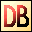DelphiBasics
 IncHourFunction Increments a TDateTime variable by + or - number of hours SysUtils unit
 function IncHour(const StartDateTime TDateTime {; NumberOfHours Integer = 1}):TDateTime;
 Description The IncHour function returns a TDateTime value that is NumberOfHours greater than the passed StartDateTime value.  The year, month, day and hour values are incremented as appropriate.  The increment value is optional, being 1 by default. Notes There is no DecHour function. Instead, use IncHour with a negative increment.Download this web site as a Windows program.

 Example code : A simple example of increment and decrement var   myDate : TDateTime; begin   // Set up our date just before the end of the year 2000   myDate := EncodeDateTime(2000, 12, 31, 23, 0, 0, 0);   ShowMessage('myDate = '+DateTimeToStr(myDate));   // Add 10 hours to this date   myDate := IncHour(myDate, 10);   ShowMessage('myDate + 10 hours = '+DateTimeToStr(myDate));   // Subtract 10 hours from this date   myDate := IncHour(myDate, -10);   ShowMessage('myDate - 10 hours = '+DateTimeToStr(myDate)); end; Show full unit code myDate = 31/12/2000 23:00:00   myDate + 10 hours = 01/01/2001 10:00:00   myDate - 10 hours = 01/01/2001 13:00:00